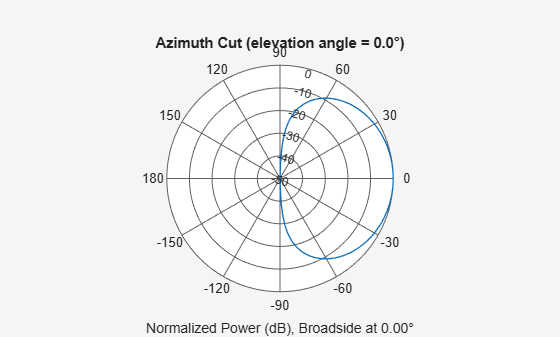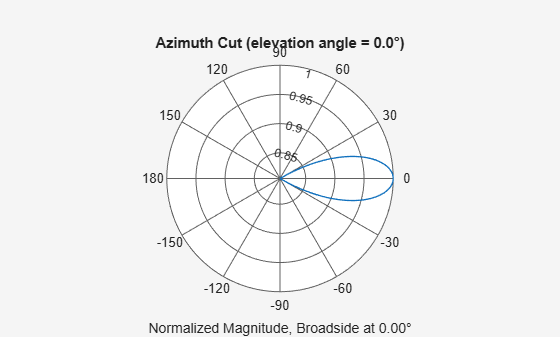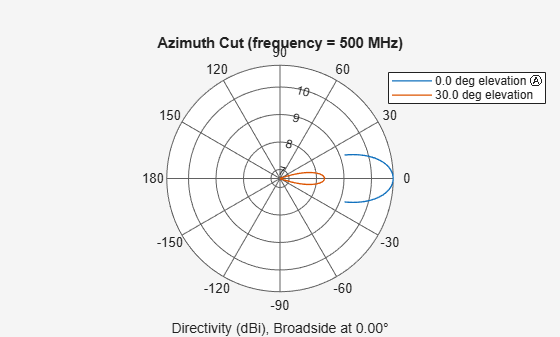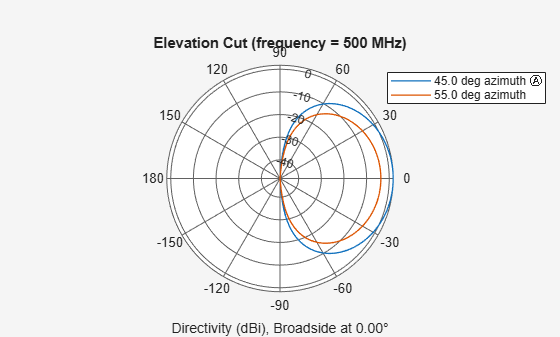# phased.CosineAntennaElement

Cosine antenna element

## Description

The `CosineAntennaElement` object models an antenna with a cosine response in both azimuth and elevation. The main response axis (MRA) points to 0° azimuth and 0° elevation in the antenna coordinate system. When placed in a linear array, the MRA is normal to the array axis (see, for example, `phased.ULA`). When placed in a planar array, the MRA points along the array normal (see, for example, `phased.URA`).

To compute the response of the antenna element for specified directions:

1. Create the `phased.CosineAntennaElement` object and set its properties.

2. Call the object with arguments, as if it were a function.

This antenna element does not support polarization.

## Creation

### Syntax

``antenna = phased.CosineAntennaElement``
``antenna = phased.CosineAntennaElement(Name,Value)``

### Description

````antenna = phased.CosineAntennaElement` creates a cosine antenna System object™, `antenna`. This object models an antenna element whose response is a cosine function raised to nonnegative powers in the azimuth and elevation directions.```

example

````antenna = phased.CosineAntennaElement(Name,Value)` creates a cosine antenna object, `antenna`, with each specified property set to the specified value. You can specify additional name-value pair arguments in any order as (`Name1`,`Value1`,...,`NameN`,`ValueN`).```

## Properties

expand all

Unless otherwise indicated, properties are nontunable, which means you cannot change their values after calling the object. Objects lock when you call them, and the `release` function unlocks them.

If a property is tunable, you can change its value at any time.

Operating frequency range of the antenna, specified as a nonnegative, real-valued, 1-by-2 row vector in the form `[LowerBound HigherBound]`. The antenna element has no response outside the specified frequency range. Units are in Hz.

Data Types: `double`

Exponents of the cosine pattern, specified as a non-negative scalar or a non-negative, real-valued, 1-by-2 vector. Exponent values must be real numbers greater than or equal to zero. When you set `CosinePower` to a scalar, both the azimuth direction cosine pattern and the elevation direction cosine pattern are raised to the same power. When you set `CosinePower` to a 1-by-2 vector, the first element is the exponent for the azimuth direction cosine pattern. The second element is the exponent for the elevation direction cosine pattern.

Example: `[1.5 1.3]`

Data Types: `double`

## Usage

### Syntax

``RESP = antenna(FREQ,ANG)``

### Description

example

````RESP = antenna(FREQ,ANG)` returns the antenna voltage response `RESP` at operating frequencies specified in `FREQ` and directions specified in `ANG`.```

Note

The object performs an initialization the first time the object is executed. This initialization locks nontunable properties and input specifications, such as dimensions, complexity, and data type of the input data. If you change a nontunable property or an input specification, the System object issues an error. To change nontunable properties or inputs, you must first call the `release` method to unlock the object.

### Input Arguments

expand all

Operating frequency of the antenna element, specified as a nonnegative scalar or nonnegative, real-valued 1-by-L row vector. Frequency units are in Hz.

`FREQ` must lie within the range of values specified by the `FrequencyRange` or the `FrequencyVector` property of the element. Otherwise, the element produces no response and the response is returned as `–Inf`. Element objects use the `FrequencyRange` property, except for `phased.CustomAntennaElement`, which uses the `FrequencyVector` property.

Example: `[1e8 2e6]`

Data Types: `double`

Azimuth and elevation angles of the response directions, specified as a real-valued 1-by-M row vector or a real-valued 2-by-M matrix, where M is the number of angular directions. Angle units are in degrees. The azimuth angle must lie in the range –180° to 180°, inclusive. The elevation angle must lie in the range –90° to 90°, inclusive.

• If `ANG` is a 1-by-M vector, each element specifies the azimuth angle of the direction. In this case, the corresponding elevation angle is assumed to be zero.

• If `ANG` is a 2-by-M matrix, each column of the matrix specifies the direction in the form `[azimuth;elevation]`.

The azimuth angle is the angle between the x-axis and the projection of the direction vector onto the xy-plane. This angle is positive when measured from the x-axis toward the y-axis. The elevation angle is the angle between the direction vector and xy-plane. This angle is positive when measured toward the z-axis. See the definition of Azimuth and Elevation Angles.

Example: `[110 125; 15 10]`

Data Types: `double`

### Output Arguments

expand all

Voltage response of antenna element, returned as a complex-valued M-by-L matrix. In this matrix, M represents the number of angles specified in `ANG` and L represents the number of frequencies specified in `FREQ`.

Data Types: `double`

## Object Functions

To use an object function, specify the System object as the first input argument. For example, to release system resources of a System object named `obj`, use this syntax:

`release(obj)`

expand all

 `beamwidth` Compute and display beamwidth of sensor element pattern `directivity` Directivity of antenna or transducer element `isPolarizationCapable` Antenna element polarization capability `pattern` Plot antenna or transducer element directivity and patterns `patternAzimuth` Plot antenna or transducer element directivity and pattern versus azimuth `patternElevation` Plot antenna or transducer element directivity and pattern versus elevation
 `step` Run System object algorithm `release` Release resources and allow changes to System object property values and input characteristics `reset` Reset internal states of System object

## Examples

collapse all

Construct a cosine antenna element and find its response in one direction. The cosine response is raised to a power of 1.5 in both azimuth and elevation. The antenna frequency range lies in the X band (from 8 to 12 GHz) at 10 GHz. Obtain the antenna's response for an incident angle of 30° azimuth and 5° elevation.

```antenna = phased.CosineAntennaElement('FrequencyRange',[8e9 12e9], ... 'CosinePower',1.5); fc = 10.0e9; ang = [30;5]; resp = antenna(fc,ang)```
```resp = 0.8013 ```

Construct a cosine pattern antenna and calculate its response at boresight (0 degrees azimuth and 0 degrees elevation). Then, plot the antenna pattern. Assume the antenna works between 800 MHz and 1.2 GHz and its operating frequency is 1 GHz. Set the azimuth exponent to 1.5 and elevation exponent to 2.5.

```antenna = phased.CosineAntennaElement('FrequencyRange',[800e6 1.2e9],... 'CosinePower',[1.5 2.5]); fc = 1e9; resp = antenna(fc,[0;0]); pattern(antenna,fc,0,-90:90,'Type','powerdb','CoordinateSystem','polar')````pattern(antenna,fc,-180:180,0,'Type','powerdb','CoordinateSystem','polar')`Construct a cosine antenna element using default parameters. Assume the antenna operating frequency is 1 GHz. Then, plot the antenna response in 3-D polar format.

```antenna = phased.CosineAntennaElement; fc = 1e9; pattern(antenna,fc,[-180:180],[-90:90],'Type','powerdb', ... 'CoordinateSystem','polar')```Compute the directivity of a cosine antenna element at seven azimuth directions centered around boresight (zero degrees azimuth and zero degrees elevation). All elevation angles are set to zero degrees.

Create a cosine antenna element system object with the `CosinePower` exponents set to 1.8.

`antenna = phased.CosineAntennaElement('CosinePower',[1.8,1.8]);`

Set the directivity angles so that the elevation angles are zero. Set the frequency to 1 GHz.

```ang = [-30,-20,-10,0,10,20,30; 0,0,0,0,0,0,0]; freq = 1e9;```

Compute the directivity.

`d = directivity(antenna,freq,ang)`
```d = 7×1 7.3890 8.6654 9.3985 9.6379 9.3985 8.6654 7.3890 ```

The maximum directivity is at boresight.

Construct a cosine antenna element using default parameters. Then, plot the pattern of the field magnitude. Assume the antenna operating frequency is 1 GHz. Restrict the response to the range of azimuth angles from -30 to 30 degrees in 0.1 degree increments. The default elevation angle is 0 degrees.

```antenna = phased.CosineAntennaElement; fc = 1e9; pattern(antenna,fc,[-30:0.1:30],0,'Type','efield', ... 'CoordinateSystem','polar')```Construct a cosine-pattern antenna. Assume the antenna works between 1 and 2 GHz and its operating frequency is 1.5 GHz. Set the azimuth angle cosine power to 2.5 and the elevation angle cosine power to 3.5. Then, plot an elevation cut of its directivity.

```antenna = phased.CosineAntennaElement('FrequencyRange', ... [1e9 2e9],'CosinePower',[2.5,3.5]); fc = 1.5e9; pattern(antenna,fc,0,-90:90,'Type','directivity', ... 'CoordinateSystem','rectangular')```The directivity is maximum at 0 degrees elevation and attains a value of approximately 12 dB.

Plot constant-elevation azimuth directivity patterns of a cosine antenna element at 0 degrees and 10 degrees elevation. Assume the operating frequency is 500 MHz.

```fc = 500e6; antenna = phased.CosineAntennaElement('FrequencyRange',[100,900]*1e6, ... 'CosinePower',[3,2]); patternAzimuth(antenna,fc,[0 30])```Plot a limited range of azimuth angles by specifying the `Azimuth` parameter. Note the change in scale.

`patternAzimuth(antenna,fc,[0 30],'Azimuth',-20:20)`Plot constant-azimuth elevation directivity patterns of a cosine antenna element at 45 and 55 degrees azimuth. Assume the operating frequency is 500 MHz.

```fc = 500e6; antenna = phased.CosineAntennaElement('FrequencyRange',[100,900]*1e6, ... 'CosinePower',[3,2]); patternElevation(antenna,fc,[45 55])```Plot a limited range of elevation angles using the Elevation parameter. Note the change in scale.

`patternElevation(antenna,fc,[45 55],'Elevation',-20:20)`Create a cosine antenna element using the `phased.CosineAntennaElement` System object™ and show that it does not support polarization.

```antenna = phased.CosineAntennaElement('FrequencyRange',[1.0,10]*1e9); isPolarizationCapable(antenna)```
```ans = logical 0 ```

The returned value `0` shows that the antenna element does not support polarization.

expand all

## Extended Capabilities

Introduced in R2011a